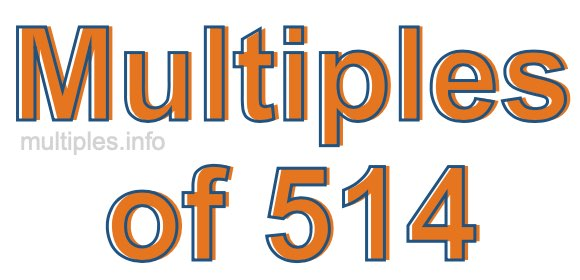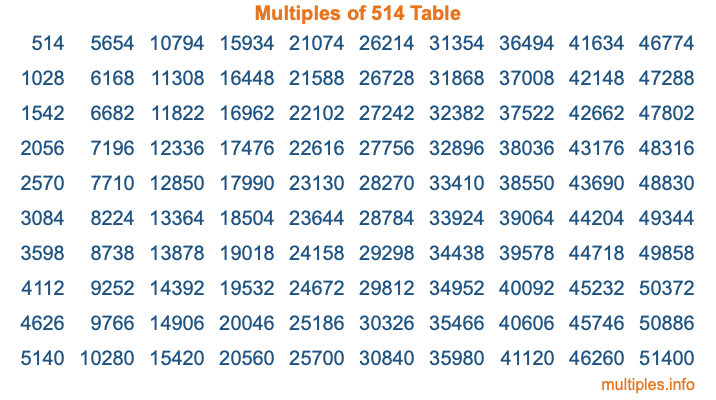Multiples of 514Welcome to the Multiples of 514 page. Here we will first teach you everything you will ever need to know about the multiples of 514, and then give you a study guide summary of everything we taught you to make sure you remember it all. Use this page to look up facts and learn information about the multiples of 514. This page will make you a multiples of five hundred fourteen expert!

Definition of Multiples of 514
Multiples of 514 are all the numbers that when divided by 514 equal an integer. Each of the multiples of 514 are called a multiple. A multiple of 514 is created by multiplying 514 by an integer.

Therefore, to create a list of multiples of 514, you start with 1 multiplied by 514, then 2 multiplied by 514, then 3 multiplied by 514, and so on for as long as you want. Thus, the list of the first five multiples of 514 is 514, 1028, 1542, 2056, and 2570. To see a larger list of multiples of 514, see the printable image of Multiples of 514 further down on this page. We also have a category where you can choose any nth multiple of 514.

Multiples of 514 Checker
The Multiples of 514 Checker below checks to see if any number of your choice is a multiple of 514. In other words, it checks to see if there is any number (integer) that when multiplied by 514 will equal your number. To do that, we divide your number by 514. If the the quotient is an integer, then your number is a multiple of 514.

Is  a multiple of 514?

Least Common Multiple of 514 and ...
A Least Common Multiple (LCM) is the lowest multiple that two or more numbers have in common. This is also called the smallest common multiple or lowest common multiple and is useful to know when you are adding our subtracting fractions. Enter one or more numbers below (514 is already entered) to find the LCM.

Check out our LCM Calculator if you need more details about the Least Common Multiple or if you need the LCM for different numbers for adding and subtraction fractions.

nth Multiple of 514
As we stated above, 514 is the first multiple of 514, 1028 is the second multiple of 514, 1542 is the third multiple of 514, and so on. Enter a number below to find the nth multiple of 514.

th multiple of 514

Multiples of 514 vs Factors of 514
514 is a multiple of 514 and a factor of 514, but that is where the similarities end. All postive multiples of 514 are 514 or greater than 514. All positive factors of 514 are 514 or less than 514.

Below is the beginning list of multiples of 514 and the factors of 514 so you can compare:

Multiples of 514: 514, 1028, 1542, 2056, 2570, etc.

Factors of 514: 1, 2, 257, 514

As you can see, the multiples of 514 are all the numbers that you can divide by 514 to get a whole number. The factors of 514, on the other hand, are all the whole numbers that you can multiply by another whole number to get 514.

It's also interesting to note that if a number (x) is a factor of 514, then 514 will also be a multiple of that number (x).

Multiples of 514 vs Divisors of 514
The divisors of 514 are all the integers that 514 can be divided by evenly. Below is a list of the divisors of 514.

Divisors of 514: 1, 2, 257, 514

The interesting thing to note here is that if you take any multiple of 514 and divide it by a divisor of 514, you will see that the quotient is an integer.

Multiples of 514 Table
Below is an image of the first 100 multiples of 514 in a table. The table is in chronological order, column by column. The first column has the first ten multiples of 514, the second column has the next ten multiples of 514, and so on.The Multiples of 514 Table is also referred to as the 514 Times Table or Times Table of 514. You are welcome to print out our table for your studies.

Negative Multiples of 514
Although not often discussed or needed in math, it is worth mentioning that you can make a list of negative multiples of 514 by multiplying 514 by -1, then by -2, then by -3, and so on, to get the following list of negative multiples of 514:

-514, -1028, -1542, -2056, -2570, etc.

Multiples of 514 Summary
Below is a summary of important Multiples of 514 facts that we have discussed on this page. To retain the knowledge on this page, we recommend that you read through the summary and explain to yourself or a study partner why they hold true.

There are an infinite number of multiples of 514.

A multiple of 514 divided by 514 will equal a whole number.

514 divided by a factor of 514 equals a divisor of 514.

The nth multiple of 514 is n times 514.

The largest factor of 514 is equal to the first positive multiple of 514.

514 is a multiple of every factor of 514.

514 is a multiple of 514.

A multiple of 514 divided by a divisor of 514 equals an integer.

514 divided by a divisor of 514 equals a factor of 514.

Any integer times 514 will equal a multiple of 514.

Multiples of a Number
Here you can get the multiples of another number, all with the same attention to detail as we did for multiples of 514 on this page.

Multiples of
Multiples of 515
Did you find our page about multiples of five hundred fourteen educational? Do you want more knowledge? Check out the multiples of the next number on our list!

Copyright  |   Privacy Policy  |   Disclaimer  |   Contact